Home | | Maths 3rd Std | Patterns in shapes

# Patterns in shapes

Creating patterns of regular and irregular shapes by stamping.

Patterns in shapes

Creating patterns of regular and irregular shapes by stamping.

Example : block patterns created using handprints and foot prints are shown below.Activity 1

Create patterns by impressing the following on a paper.

(i) Using dry leaves /fallen leaves.

(ii) Fingers, hands, toes, feet.

(iii) Using bangles.Activity 2

Create patterns on your own by impressing the following on a chart paper and decorate your class room.

i. seeds

ii. buttons

iii. bottle lids

My own patternsPattern in geometrical shapes

There are two types of patterns.

They are

Growing patterns.

Repeated patterns.

a. Growing Patterns

If some patterns and designs increase or grow with straight lines and geometrical forms, they are called growing patterns.

Example :Try this

Create some growing patterns using circle and square.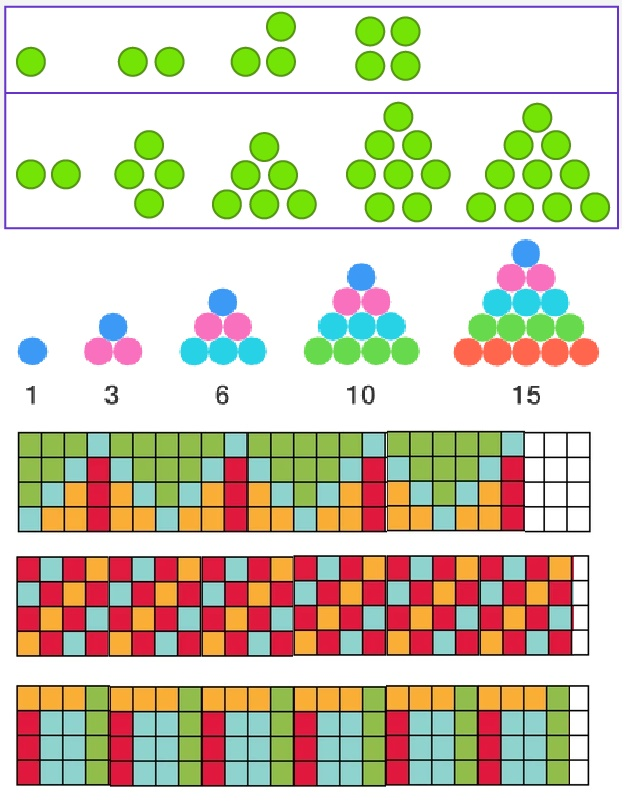Practice

a. Continue the growing patterns.b. Continue the growing pattern.b. Repeated Patterns

If some patterns and designs repeat with straight lines and geometrical shapes, they are called repeated patterns.

Example :Practice

Continue the repeating patterns upto 3 steps in the space provided.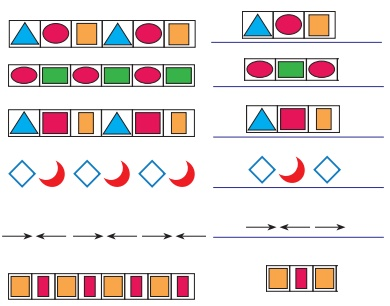Activity 3

Complete the buntings by following the pattern.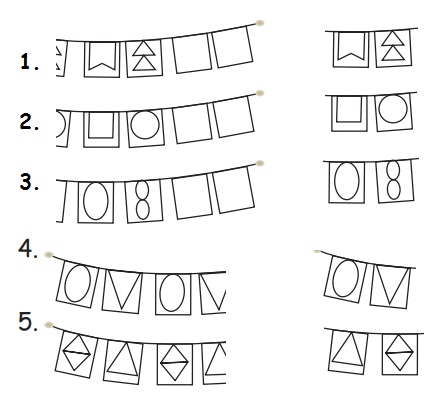Do yourself: Draw some repeated patterns of your own.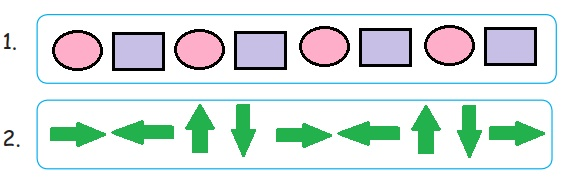Patterns are created by combining colours and shapes in different ways.Activity 4

1. Match the following and complete the pattern.2. Match the following.Symmetries in shapes and patterns.1. Take a piece of paper.

2. Spill few drops of ink on the paper.

3. Now fold the paper and press it.

4. You will get a symmetric figure.

Symmetry

Definition

Symmetry means that one shape becomes exactly like another when you move it in the some way: turn, flip or slide.

Let us do1. Take a sheet of paper and fold it.

2. Draw any shape at the folded edge of the sheet.

3. Cut the shape

4. Unfold it

Example :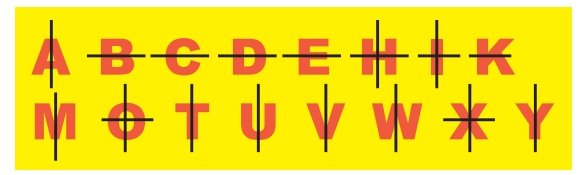line of symmetry

Note that one half of the shape is exactly like the other half. The line which divides the figure into two exact halves is called the line of symmetry.

The following letters are non symmetric and does not have line of symmetry.F G J L N P Q R S Z

Try this

Make the paper cut outs of the shapes shown below with the help of your elders and keep them in in front of a mirror and observe the image formed in the mirror. You could see the other half of the image.Symmetrical shapes

Definition

If a shape can be folded or divided into half so that the two halves match exactly then such a shape is called a symmetric shapes.

Example : 1Example : 2

Line of Symmetry, Folding Line, Mirror Line, Mirror LineProject:

Collect some symmetrical images from newspapers and magazines and Paste them to make an album.

Activity 5

Complete the symmetrical imageTeacher’s note : Teacher can guide the children to collect the symmetrical images in day−today life.

Activity 6

a. Draw the lines of symmetry for the following figures.b. Circle the non−symmetrical shapes.Activity 7

Draw the other half from the line of symmetry to make it symmetrical.Activity 8

Line symmetry is also found in some letters of the alphabet. Complete, Write the letters with line symmetry.

Answer: A B C D E H I K M O T U V W X Y Z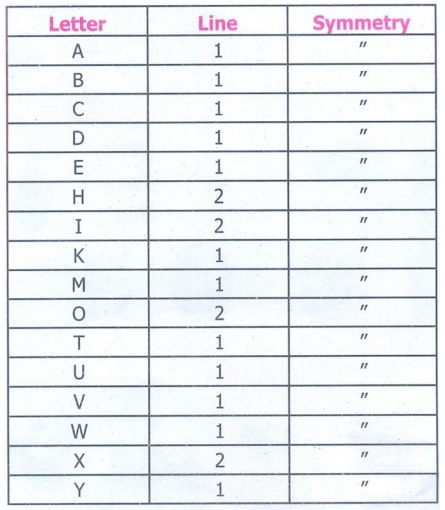Tags : Patterns | Term 1 Chapter 3 | 3rd Maths , 3rd Maths : Term 1 Unit 3 : Patterns
Study Material, Lecturing Notes, Assignment, Reference, Wiki description explanation, brief detail
3rd Maths : Term 1 Unit 3 : Patterns : Patterns in shapes | Patterns | Term 1 Chapter 3 | 3rd Maths